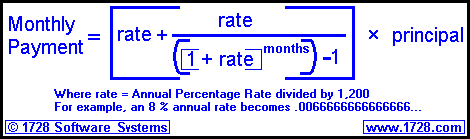Loan Payment FormulaIf we borrow \$150,000 for 30 years at 8 per cent annual percentage rate. What is the monthly payment? 1) The rate would be 8 divided by 1,200       which equals .0066666666... 2) The months would be 12 × 30 = 360. 3) So the monthly Payment would be:
 .0066666666         .0066666666   +   ((1.0066666666 ^360)-1)       ×   \$150,000 4) The middle section of the equation is the most difficult to solve so we will calculate that first. (.0066666666) ÷ ((1.0066666666 ^360)-1) = (.0066666666) ÷ (10.935729397036 -1) = (.0066666666) ÷ 9.935729397036 = 0.0006709790897 5) "Plugging" this into the rest of the equation we have: (.0066666666   +   0.0006709790897) × \$150,000 = (0.007337645756) × \$150,000 = \$ 1,100.65 Aren't you glad you have the Loan Calculator to use instead of this method?

RETURN   TO   HOME   PAGE